# Volume

Here you will learn about volume, including what it is and how to calculate it to solve problems.

Students will first learn about volume as part of measurement and data in the 5 th grade, and continue to expand on their knowledge in geometry in the 6 th and 7 th grade.

## What is volume?

Volume is a measurement of the amount of space there is within 3D shapes.

To calculate the volume of an object, there are various volume formulas.

Cube \text { Volume }=a^3
Rectangular prism \text{Volume}=l\times w\times h
Prism \text{Volume}=\text{Area of base} \times \text{Length}
Cylinder \text{Volume}=\pi r^2h

(\text { Volume }=\text { Area of base } \times \text { Height } )
Pyramid\text{Volume}=\cfrac{1}{3} \, \times \text{Area of base} \times \text{Height}
Cone \text{Volume}=\cfrac{1}{3} \, \pi r^2h

(\text{Volume}=\cfrac{1}{3} \, \times \text{Area of base} \times \text{Height})
Sphere \text{Volume}=\cfrac{4}{3} \, \pi r^3

Step-by-step guide: Volume formula

Let’s look at each type of 3 -dimensional shape.

A prism is made up of 2 congruent bases, connected by lateral faces. For right prisms, the lateral sides are always rectangles.

For example,

Imagine filling this triangle prism fully with water. The total amount of water inside the prism would represent the volume of the prism in cubic units.

\text { Volume of prism }=\text { Area of the base } \times \text { height }

To calculate the volume of any prism, calculate the area of the base and times it by the height.

Step-by-step guide: Volume of a prism

The volume of a rectangular prism can be found by counting the cubic units with the prism.

This rectangular prism is made from 24 unit cubes – each side is 1 \, cm. That means the space within the rectangular prism, or the volume, is 24 \, cm^3.

You can prove that the volume is 24 \, cm^3 , by counting the total cubes, or by using the formula.

\begin{aligned}\text { Volume of a rectangular prism } & =\text { length } \times \text { width } \times \text { height } \\\\ & =6 \mathrm{~cm} \times 2 \mathrm{~cm} \times 2 \mathrm{~cm} \\\\ & =24 \mathrm{~cm}^3\end{aligned}

Step-by-step guide: Volume of a rectangular prism

To find the volume of a cube, use the volume of a cube formula. The formula is similar to l \times w \times h , but since all sides of a cube are the same length, you can just multiply one side length, a , three times: \text {Volume }=a^{3} .

For example,

The volume of this cube is:

\begin{aligned}& \text { volume }=a^3 \\\\ & \text { volume }=5^3 \\\\ & \text { volume }=125 \mathrm{~ft}^3\end{aligned}

Step-by-step guide: Volume of a cube

Step-by-step guide: Volume of a pyramid

### What is volume?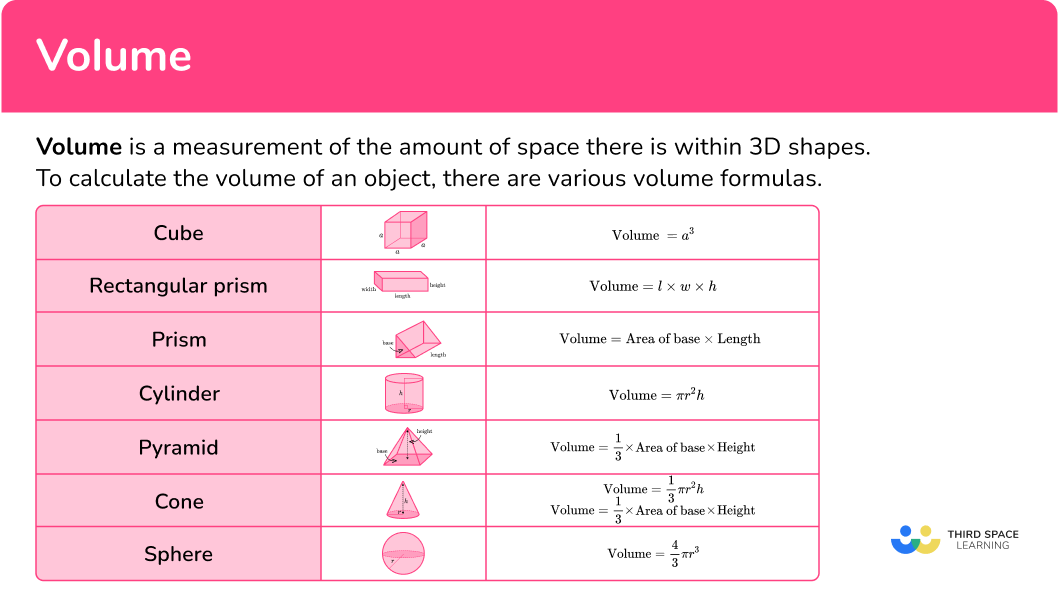## Common Core State Standards

How does this relate to 5 th, 6 th and 7 th grade math?

• Grade 5: Measurement and Data (5.MD.C.5b)
Apply the formulas V=l \times w \times h and V=b \times h for rectangular prisms to find volumes of right rectangular prisms with whole number edge lengths in the context of solving real world and mathematical problems.

Find the volume of a right rectangular prism with fractional edge lengths by packing it with unit cubes of the appropriate unit fraction edge lengths, and show that the volume is the same as would be found by multiplying the edge lengths of the prism.

Apply the formulas V=l \times w \times h and V=b \times h to find volumes of right rectangular prisms with fractional edge lengths in the context of solving real-world and mathematical problems.

Solve real-world and mathematical problems involving area, volume and surface area of two- and three-dimensional objects composed of triangles, quadrilaterals, polygons, cubes, and right prisms.

## How to calculate volume

In order to calculate volume:

1. Write down the formula.
2. Substitute the values into the formula.
3. Calculate the volume of the shape.
4. Write the answer, including the units.

## Volume formula examples

### Example 1: volume of a triangular prism

Calculate the volume of the triangular prism:

1. Write down the formula.

\text { Volume of prism }=\text { Area of the base } \times \text { height }

2Substitute the values into the formula.

A triangular prism has two congruent triangular bases connected by lateral faces – so the base is the triangle. The height of the prism is 7 \, cm.

\begin{aligned}\text { Volume of prism }&=\text { Area of the base (triangle }) \, \times \, \text { height of prism } \\\\ & =\cfrac{1}{2} \times 3 \times 4 \times \, 7 \\\\ &\end{aligned}

3Calculate the volume of the shape.

\begin{aligned}\text { Volume } & =\cfrac{1}{2} \, \times 3 \, \times 4 \, \times 7 \\\\ & =6 \, \times \, 7 \\\\ & =42\end{aligned}

4Write the answer, including the units.

The measurements on this triangular prism are in centimeters, so the volume is measured in cubic centimeters.

\text { Volume }=42 \mathrm{~cm}^3

### Example 2: volume of a cube – fractions

Calculate the volume of the cube:

\text { Volume of a cube }=a^3

A cube has a side length of 3 \cfrac{4}{5} , so a=3 \cfrac{4}{5} .

\begin{aligned} \text { Volume of a cube } & =a^3 \\\\ & =\left(3 \cfrac{4}{5}\right)^3 \\\\ \end{aligned}

\begin{aligned} \text { Volume } & =3 \cfrac{4}{5} \, \times \, 3 \cfrac{4}{5} \, \times \, 3 \cfrac{4}{5} \\\\ & =14 \cfrac{11}{25} \, \times \, 3 \cfrac{4}{5} \\\\ & =\cfrac{361}{25} \, \times \, \cfrac{19}{5} \\\\ & =\cfrac{6,859}{125} \\\\ & =54 \cfrac{109}{125}\end{aligned}

The measurements on this cube are in meters, so the volume is measured in cubic meters.

\text { Volume }=54 \cfrac{109}{125} \mathrm{~m}^3

### Example 3: volume of a pentagonal prism

Calculate the volume of the prism:

\text { Volume of prism }=\text { Area of the base } \times \text { height }

A pentagonal prism has two congruent pentagonal bases connected by lateral faces – so the base is a pentagon. The area of the pentagon is 15 \, ft^2. The height of the prism is 9 \, ft .

\begin{aligned} \text { Volume of prism } & =\text { Area of the base (pentagon}) \, \times \, \text {height of prism } \\\\ & = 15 \, \times \, 9\\\\ \end{aligned}

\begin{aligned}\text {Volume of prism } & =15 \, \times \, 9 \\\\ & =135\end{aligned}

The measurements on this prism are in feet, so the volume is measured in cubic feet.

\text { Volume }=135 \mathrm{ft}^3

### Example 4: missing dimension

The volume of the rectangular prism is 108 \mathrm{~cm}^3.

Calculate the value of x .

The 3D shape is a rectangular prism.

The formula you need to use is \text{Volume}=l\times w\times h.

The length (l), the width (w) and the height (h) of the rectangular prism are interchangeable, so it doesn’t matter which dimension l or w or h.

The values you need to substitute into the formula are \text {Volume}=108, l=4 and w=4.5.

For the height (h) , you can use x .

\begin{aligned}\text { Volume } &=l \, \times \, w \, \times \, h \\\\ \ 108 &=4 \, \times \, 4.5 \, \times \, x \\\\ \ 108 &=18 \, \times \, x\end{aligned}

In this case, you already know the volume. You divide the volume by the area of the base to find the missing height.

x=108 \div 18=6.

The dimensions of the rectangular prism were given in centimeters, and the volume was given in cubic centimeters (cm^3).

Since x is a dimension of the rectangular prism, it is recorded in just units, not units cubed.

x=6 \, cm

### Example 5: missing dimension

The volume of the square pyramid is 32 \text { inches }^3.

Calculate the height of the pyramid.

The 3D shape is a pyramid. The formula you need to use is:

\text{Volume}=\cfrac{1}{3} \, \times \, \text{Area of Base} \times \text{Height}

First you need to calculate the area of the square base, 64=4 \times 4=16.

The values you need to substitute into the formula are \text{volume}=32 and \text{area of base}=16.

For the height, you can use h .

\begin{aligned}\text{Volume} &=\cfrac{1}{3} \, \times \, \text{Area of base} \, \times \, \text{Height}\\\\ 32 & =\cfrac{1}{3} \, \times \, 16 \times \, h \\\\ 32 &=5 \cfrac{1}{3} \, \times \, h\end{aligned}

In this case, you already know the volume. You divide the volume by the area of the base to find the missing height.

\begin{aligned}h & =32 \div 5 \cfrac{1}{3} \\\\ & =32 \div \cfrac{16}{3} \\\\ & =\cfrac{32}{1} \times \cfrac{3}{16} \\\\ & =\cfrac{96}{16} \\\\ & =6\end{aligned}

The dimensions of the pyramid were given in inches and the volume was given in cubic inches (inches^3).

Since h is a dimension of the square pyramid, it is recorded in just units, not units cubed.

h=6 \, inches

### Example 6: volume of a compound shape

Calculate the volume of the compound 3D shape.

The 3D shape is made from a rectangular prism and a pyramid. Find the volume of each part and then add the volumes together. Use the formulas:

The volume of the shape is:

\text { Total Volume }=1,694+363=2,057.

The dimensions of the compound 3D shape are in centimeters, so the volume is in cubic centimeters (cm^3).

V=2,057 \, cm^3

### Teaching tips for volume

• Have students create or identify examples of cubic units to be displayed in the classroom. For example, most ones cubes included in math manipulative kits are 1 centimeter cubed. Students can take 12 rulers and tape them together to form a cubic foot, 12 yard sticks to form a cubic yard or 12 meter sticks to form a cubic meter.

Activities like this give students a visual understanding of how big or small different cubic units are and can also lead to discussions about why labeling cubic units is important.

• Incorporate interactive demonstrations to show what volume is and why the formulas work. For example, using the volume of a container. Start by filling the container with water and asking students to estimate the volume of water within it.

Then measure the volume of water in mL and use the dimensions of the container to calculate the volume in centimeters cubed. Use the findings or student thinking to guide a conversation about what this activity shows us about volume.

• Uses quizzes, worksheets, or project assignments to provide timely and constructive feedback to address any misconceptions or errors when calculating volume or using the volume formulas.

### Easy mistakes to make

• Using the incorrect units
Volume is always measured in cubic units. Not including units or including the incorrect units does not accurately represent volume as a measurement.
For example,
The volume of the following shape is measured in cubic inches, since the side lengths are measured in inches.

• Using the volume formula with different units
All measurements of a shape being used in the volume formula need to be in the same units before calculating the volume.

• Confusing the formulas for calculating volume
There are many different formulas used for volume, and it is easy to get them confused. Always think about whether or not the formula you are using makes sense.
For example,
The formula for the volume of a triangular prism is \cfrac{1}{2} \times b \times h \times p r i s m \text { height }.
Within the formula is the area of a triangle (\cfrac{1}{2} \times b \times h), which is the base and then that base area times the height. If you wanted to find the area of a rectangular prism, you should recognize that there is no triangle, so instead the area of the base would need to be l \times w .

### Practice volume questions

1. Calculate the volume of this pyramid.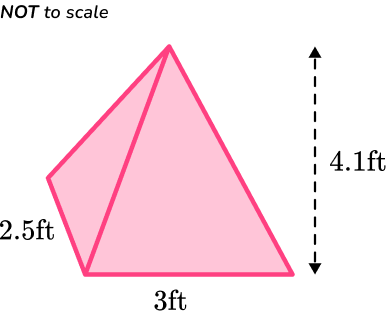30.75 \text { inches}^315.375 \text { inches}^331.27 \text { inches}^310. 25 \text { inches}^3The volume formula to calculate the volume of the pyramid is:

\text{Volume} =\cfrac{1}{3}\times \text{Area of base}\times \text{Height}.

You need to find the area of the base of the pyramid.

A=2.5 \times 3=7.5

\begin{aligned} \text{ Volume }&=\cfrac{1}{3} \times \text { Area of base } \times \text { Height } \\\\ & =\cfrac{1}{3} \times 7.5 \times 4.1 \\\\ & =10.25\end{aligned}

The volume of the pyramid is 10.25 \text { inches}^3.

2. The volume of this rectangular prism is 780 \mathrm{~mm}^3. Calculate the length of the missing side.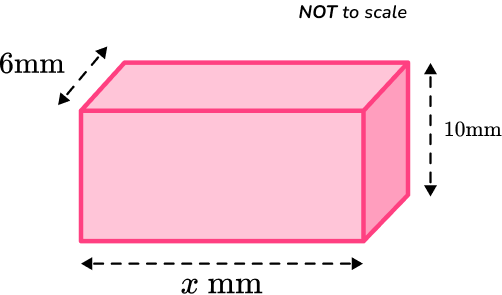13 \, mm13 \, cm15 \, mm15 \, cmThe volume formula to calculate the volume of the rectangular prism is  \text{Volume} =l\times w\times h.

You need to substitute the values given, using x for the unknown length, into the volume formula.

\begin{aligned}\text { Volume }&=l \times w \times h \\\\ 780 &=x \times 6 \times 10 \\\\ 780 &=x \times 60\end{aligned}

The missing length is x=780 \div 60=13.

The missing length of the rectangular prism is 13 \, mm.

3. Calculate the volume of this triangular prism.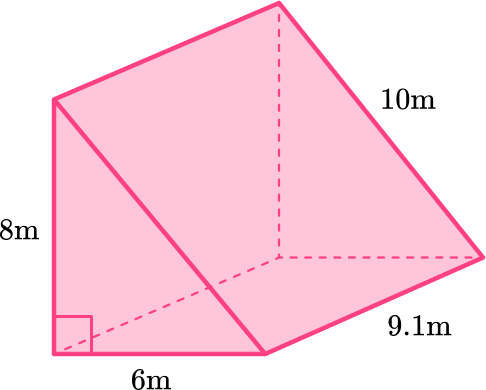2,184 \mathrm{~m}^34,368 \mathrm{~m}^3436.8 \mathrm{~m}^3218.4 \mathrm{~m}^3A triangular prism has two congruent triangular bases connected by lateral faces – so the base is the triangle.

\begin{aligned}\text { Area of triangle } &=\cfrac{1}{2} \times b \times h \\\\ &=\cfrac{1}{2} \times 6 \times 8 \\\\ &=24\end{aligned}

The area of the triangle is 24 \mathrm{~m}^2.

The height of the prism is 9.1 \, m.

\begin{aligned}\text { Volume of prism } & =\text { Area of the base } \times \text { height } \\\\ & =24 \times 9.1 \\\\ & =218.4\end{aligned}

The measurements on this triangular prism are in meters, so the volume is measured in cubic meters.

\text { Volume }=218.4 \mathrm{~m}^3

4. Calculate the volume of this hexagonal prism.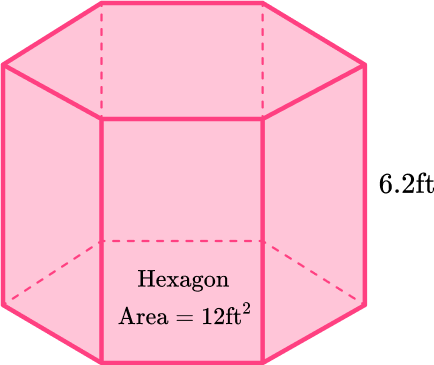98.4 \mathrm{ft}^361.2 \mathrm{ft}^337.2 \mathrm{ft}^374.4 \mathrm{ft}^3Calculate the volume of the prism:

\text { Volume of prism }=\text { Area of the base } \times \text { height }

A hexagonal prism has two congruent hexagonal bases connected by lateral faces – so the base is a hexagon. The area of the hexagon is 12 \mathrm{ft}^2.

The height of the prism is 6.2 \, ft.

\begin{aligned}\text { Volume of prism } & =\text { Area of the base } \times \text { height } \\\\ & =12 \times 6.2 \\\\ & =74.4\end{aligned}

The measurements on this prism are in feet, so the volume is measured in cubic feet.

\text { Volume }=74.4 \mathrm{ft}^3

5. Calculate the volume of this triangular prism.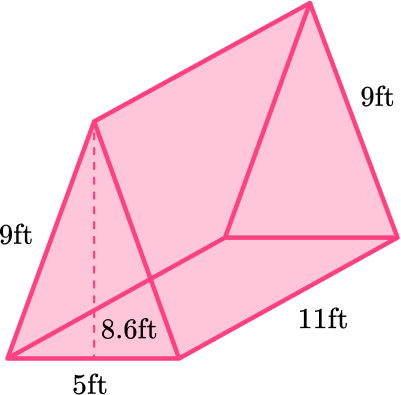473 \mathrm{ft}^34,730 \mathrm{ft}^32,365 \mathrm{ft}^3236.5 \mathrm{ft}^3A triangular prism has two congruent triangular bases connected by lateral faces – so the base is the triangle.

\begin{aligned}\text { Area of triangle } & =\cfrac{1}{2} \times b \times h \\\\ & =\cfrac{1}{2} \times 5 \times 8.6 \\\\ & =21.5\end{aligned}

The area of the triangle is 21.5 \mathrm{ft}^2.

The height of the prism is 11 \, ft.

\begin{aligned}\text { Volume of prism } & =\text { Area of the base } \times \text { height } \\\\ &=21.5 \times 11 \\\\ & =236.5\end{aligned}

The measurements on this triangular prism are in feet, so the volume is measured in cubic feet.

\text { Volume }=236.5 \mathrm{ft}^3

6. Calculate the volume of the shape below.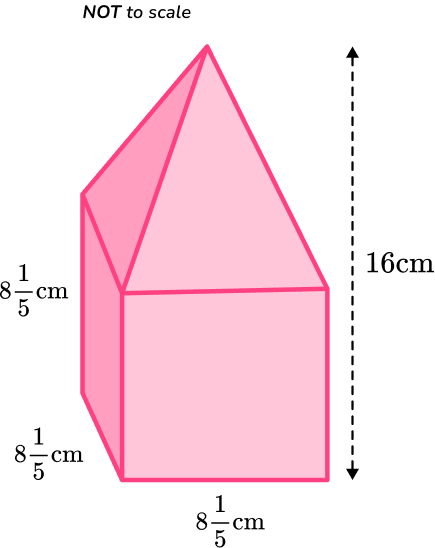8,821 \, \cfrac{111}{125} \mathrm{~cm}^3551 \, \cfrac{46}{125} \mathrm{~cm}^3726 \, \cfrac{24}{125} \mathrm{~cm}^3174 \, \cfrac{309}{375} \mathrm{~cm}^3The volume of the rectangular prism is:

\begin{aligned} \text { Volume }&=l \times w \times h \\\\ & =8 \, \cfrac{1}{5} \, \times 8 \, \cfrac{1}{5} \, \times 8 \, \cfrac{1}{5} \\\\ & =\cfrac{41}{5} \, \times \cfrac{41}{5} \, \times \cfrac{41}{5} \\\\ & =\cfrac{68,921}{125} \\\\ & =551 \, \cfrac{46}{125}\end{aligned}

The height of the pyramid is 16-8 \, \cfrac{1}{5} \, =7 \, \cfrac{4}{5}.

The volume of the pyramid is

\begin{aligned}\text { Volume } &=\cfrac{1}{3} \, \times \text { Area of base } \times \text { Height } \\\\ & =\cfrac{1}{3} \, \times 8 \, \cfrac{1}{5} \times 8 \, \cfrac{1}{5} \, \times 7 \, \cfrac{4}{5} \\\\ & =\cfrac{1}{3} \, \times \cfrac{41}{5} \, \times \cfrac{41}{5} \, \times \cfrac{39}{5} \\\\ & =\cfrac{65,559}{375} \\\\ & =174 \, \cfrac{309}{375}\end{aligned}

The total volume can be found by adding the two volumes together.

\begin{aligned}\text { Total Volume } &=551 \, \cfrac{46}{125}+174 \, \cfrac{309}{375} \\\\ & =551 \, \cfrac{138}{375}+174 \, \cfrac{309}{375} \\\\ & =725 \, \cfrac{447}{375} \\\\ & =726 \, \cfrac{72}{375}\mathrm{~cm}^3\end{aligned}

## Volume formula FAQs

How do you calculate the volume of a cuboid?

The volume of a cuboid is found by multiplying the length, width and height. It is the same as a rectangular prism, because they are the same shape – they are synonyms.

How do you calculate the volume of a cone and the volume of a cylinder?

Both of these shapes have a circular base, so you need to know how to find the area of a circle. Once you know how to find the area of a circle, you can use the following formulas to calculate the volume of each: cone is \cfrac{1}{3} \pi r^2 h and cylinder is \pi r^2 h.

How is volume different from area?

Area is a measurement of two-dimensional space and volume is a measurement of three-dimensional space inside of three-dimensional shapes.

What is the metric system?

The metric system is a system of measurements that include millimeter, centimeter, meter, kilometer and more. It is a base 10 measurement system that is used throughout the world.

## Still stuck?

At Third Space Learning, we specialize in helping teachers and school leaders to provide personalized math support for more of their students through high-quality, online one-on-one math tutoring delivered by subject experts.

Each week, our tutors support thousands of students who are at risk of not meeting their grade-level expectations, and help accelerate their progress and boost their confidence.